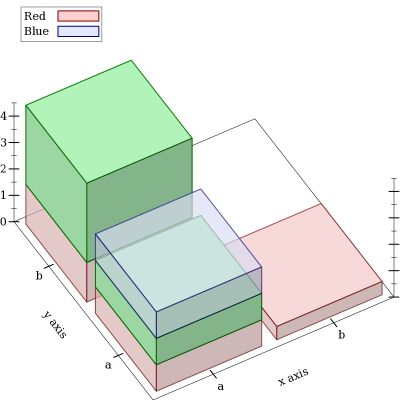Version: 5.2.1

### 53D Renderers

#### 5.13D Renderer Function Arguments

As with functions that return 2D renderers, functions that return 3D renderers always have these kinds of arguments:
• Required (and possibly optional) arguments representing the graph to plot.

• Optional keyword arguments for overriding calculated bounds, with the default value #f.

• Optional keyword arguments that determine the appearance of the plot.

• The optional keyword argument #:label, which specifies the name of the renderer in the legend.

See 2D Renderer Function Arguments for a detailed example.

#### 5.23D Point Renderers

 (points3d vs [ #:x-min x-min #:x-max x-max #:y-min y-min #:y-max y-max #:z-min z-min #:z-max z-max #:sym sym #:color color #:fill-color fill-color #:size size #:line-width line-width #:alpha alpha #:label label]) → renderer3d?
vs : (listof (vector/c real? real? real?))
x-min : (or/c rational? #f) = #f
x-max : (or/c rational? #f) = #f
y-min : (or/c rational? #f) = #f
y-max : (or/c rational? #f) = #f
z-min : (or/c rational? #f) = #f
z-max : (or/c rational? #f) = #f
sym : point-sym/c = (point-sym)
color : plot-color/c = (point-color)
fill-color : (or/c plot-color/c 'auto) = 'auto
size : (>=/c 0) = (point-size)
line-width : (>=/c 0) = (point-line-width)
alpha : (real-in 0 1) = (point-alpha)
label : (or/c string? #f) = #f
Returns a renderer that draws points in 3D space.

For example, a scatter plot of points sampled uniformly from the surface of a sphere:
> (define (runif) (- (* 2 (random)) 1))
> (define (rnormish) (+ (runif) (runif) (runif) (runif)))
> (define xs0 (build-list 1000 (λ _ (rnormish))))
> (define ys0 (build-list 1000 (λ _ (rnormish))))
> (define zs0 (build-list 1000 (λ _ (rnormish))))
 > (define mags (map (λ (x y z) (sqrt (+ (sqr x) (sqr y) (sqr z)))) xs0 ys0 zs0))
> (define xs (map / xs0 mags))
> (define ys (map / ys0 mags))
> (define zs (map / zs0 mags))
 > (plot3d (points3d (map vector xs ys zs) #:sym 'dot) #:altitude 25)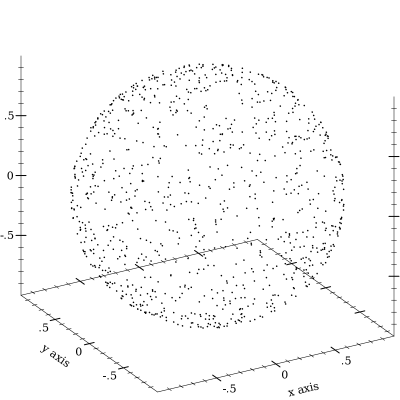(vector-field3d f [ x-min x-max y-min y-max z-min z-max #:samples samples #:scale scale #:color color #:line-width line-width #:line-style line-style #:alpha alpha #:label label]) → renderer3d?
f :
 (or/c (real? real? real? . -> . (vector/c real? real? real?)) ((vector/c real? real? real?) . -> . (vector/c real? real? real?)))
x-min : (or/c rational? #f) = #f
x-max : (or/c rational? #f) = #f
y-min : (or/c rational? #f) = #f
y-max : (or/c rational? #f) = #f
z-min : (or/c rational? #f) = #f
z-max : (or/c rational? #f) = #f
samples : exact-positive-integer? = ( vector-field3d-samples)
 scale : (or/c real? (one-of/c 'auto 'normalized)) = (vector-field-scale)
color : plot-color/c = (vector-field-color)
line-width : (>=/c 0) = (vector-field-line-width)
line-style : plot-pen-style/c = (vector-field-line-style)
alpha : (real-in 0 1) = (vector-field-alpha)
label : (or/c string? #f) = #f
Returns a renderer that draws a vector field in 3D space. The arguments are interpreted identically to the corresponding arguments to vector-field.

Example:

 > (plot3d (vector-field3d (λ (x y z) (vector x z y)) -2 2 -2 2 -2 2))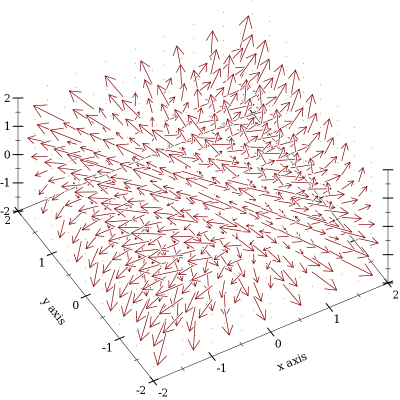#### 5.33D Line Renderers

 (lines3d vs [ #:x-min x-min #:x-max x-max #:y-min y-min #:y-max y-max #:z-min z-min #:z-max z-max #:color color #:width width #:style style #:alpha alpha #:label label]) → renderer3d?
vs : (listof (vector/c real? real? real?))
x-min : (or/c rational? #f) = #f
x-max : (or/c rational? #f) = #f
y-min : (or/c rational? #f) = #f
y-max : (or/c rational? #f) = #f
z-min : (or/c rational? #f) = #f
z-max : (or/c rational? #f) = #f
color : plot-color/c = (line-color)
width : (>=/c 0) = (line-width)
style : plot-pen-style/c = (line-style)
alpha : (real-in 0 1) = (line-alpha)
label : (or/c string? #f) = #f
Returns a renderer that draws connected lines. The parametric3d function is defined in terms of this one.

 (parametric3d f t-min t-max [ #:x-min x-min #:x-max x-max #:y-min y-min #:y-max y-max #:z-min z-min #:z-max z-max #:samples samples #:color color #:width width #:style style #:alpha alpha #:label label]) → renderer3d?
f : (real? . -> . (vector/c real? real? real?))
t-min : rational?
t-max : rational?
x-min : (or/c rational? #f) = #f
x-max : (or/c rational? #f) = #f
y-min : (or/c rational? #f) = #f
y-max : (or/c rational? #f) = #f
z-min : (or/c rational? #f) = #f
z-max : (or/c rational? #f) = #f
samples : (and/c exact-integer? (>=/c 2)) = (line-samples)
color : plot-color/c = (line-color)
width : (>=/c 0) = (line-width)
style : plot-pen-style/c = (line-style)
alpha : (real-in 0 1) = (line-alpha)
label : (or/c string? #f) = #f
Returns a renderer that plots a vector-valued function of time. For example,
> (require (only-in plot/utils 3d-polar->3d-cartesian))
 > (plot3d (parametric3d (λ (t) (3d-polar->3d-cartesian (* t 80) t 1)) (- pi) pi #:samples 3000 #:alpha 0.5) #:altitude 25)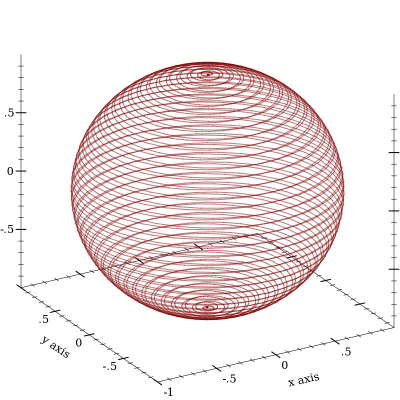#### 5.43D Surface Renderers

 (surface3d f [ x-min x-max y-min y-max #:z-min z-min #:z-max z-max #:samples samples #:color color #:style style #:line-color line-color #:line-width line-width #:line-style line-style #:alpha alpha #:label label]) → renderer3d?
f : (real? real? . -> . real?)
x-min : (or/c rational? #f) = #f
x-max : (or/c rational? #f) = #f
y-min : (or/c rational? #f) = #f
y-max : (or/c rational? #f) = #f
z-min : (or/c rational? #f) = #f
z-max : (or/c rational? #f) = #f
samples : (and/c exact-integer? (>=/c 2)) = (plot3d-samples)
color : plot-color/c = (surface-color)
style : plot-brush-style/c = (surface-style)
line-color : plot-color/c = (surface-line-color)
line-width : (>=/c 0) = (surface-line-width)
line-style : plot-pen-style/c = (surface-line-style)
alpha : (real-in 0 1) = (surface-alpha)
label : (or/c string? #f) = #f
Returns a renderer that plots a two-input, one-output function. For example,
 > (plot3d (list (surface3d (λ (x y) (+ (sqr x) (sqr y))) -1 1 -1 1 #:label "z = x^2 + y^2") (surface3d (λ (x y) (- (+ (sqr x) (sqr y)))) -1 1 -1 1 #:color 4 #:line-color 4 #:label "z = -x^2 - y^2")))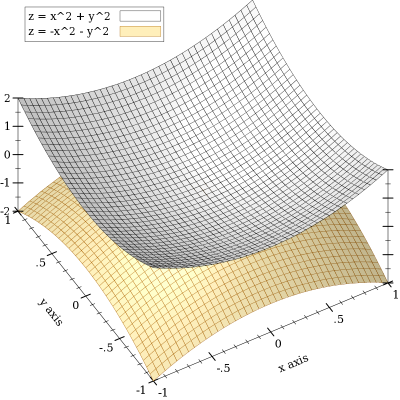(polar3d f [ #:x-min x-min #:x-max x-max #:y-min y-min #:y-max y-max #:z-min z-min #:z-max z-max #:samples samples #:color color #:style style #:line-color line-color #:line-width line-width #:line-style line-style #:alpha alpha #:label label]) → renderer3d?
f : (real? real? . -> . real?)
x-min : (or/c rational? #f) = #f
x-max : (or/c rational? #f) = #f
y-min : (or/c rational? #f) = #f
y-max : (or/c rational? #f) = #f
z-min : (or/c rational? #f) = #f
z-max : (or/c rational? #f) = #f
samples : (and/c exact-integer? (>=/c 2)) = (plot3d-samples)
color : plot-color/c = (surface-color)
style : plot-brush-style/c = (surface-style)
line-color : plot-color/c = (surface-line-color)
line-width : (>=/c 0) = (surface-line-width)
line-style : plot-pen-style/c = (surface-line-style)
alpha : (real-in 0 1) = (surface-alpha)
label : (or/c string? #f) = #f
Returns a renderer that plots a function from latitude and longitude to radius.

Currently, latitudes range from 0 to (* 2 pi), and longitudes from (* -1/2 pi) to (* 1/2 pi). These intervals may become optional arguments to polar3d in the future.

A sphere is the graph of a polar function of constant radius:
 > (plot3d (polar3d (λ (θ ρ) 1)) #:altitude 25)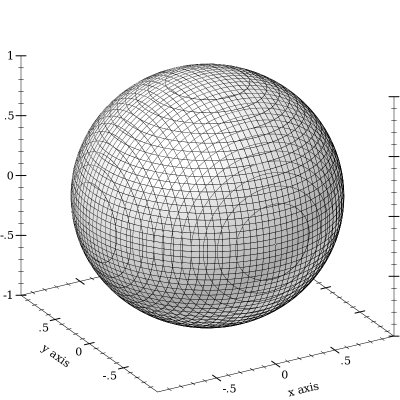Combining polar function renderers allows faking latitudes or longitudes in larger ranges, to get, for example, a seashell plot:
 > (parameterize ([plot-decorations?  #f] [plot3d-samples     75]) (define (f1 θ ρ) (+ 1 (/ θ 2 pi) (* 1/8 (sin (* 8 ρ))))) (define (f2 θ ρ) (+ 0 (/ θ 2 pi) (* 1/8 (sin (* 8 ρ))))) (plot3d (list (polar3d f1 #:color "navajowhite" #:line-style 'transparent #:alpha 2/3) (polar3d f2 #:color "navajowhite" #:line-style 'transparent #:alpha 2/3))))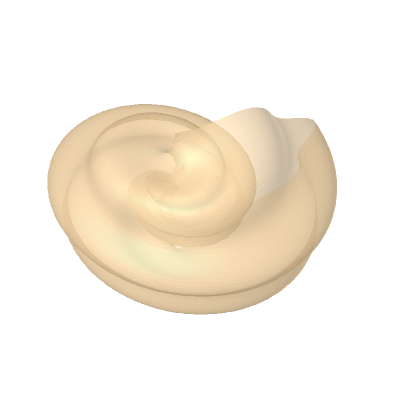#### 5.53D Contour (Isoline) Renderers

 (isoline3d f z [ x-min x-max y-min y-max #:z-min z-min #:z-max z-max #:samples samples #:color color #:width width #:style style #:alpha alpha #:label label]) → renderer3d?
f : (real? real? . -> . real?)
z : real?
x-min : (or/c rational? #f) = #f
x-max : (or/c rational? #f) = #f
y-min : (or/c rational? #f) = #f
y-max : (or/c rational? #f) = #f
z-min : (or/c rational? #f) = #f
z-max : (or/c rational? #f) = #f
samples : (and/c exact-integer? (>=/c 2)) = (plot3d-samples)
color : plot-color/c = (line-color)
width : (>=/c 0) = (line-width)
style : plot-pen-style/c = (line-style)
alpha : (real-in 0 1) = (line-alpha)
label : (or/c string? #f) = #f
Returns a renderer that plots a single contour line on the surface of a function.

The appearance keyword arguments are interpreted identically to the appearance keyword arguments to isoline.

This function is not terribly useful by itself, but can be when combined with others:
> (define (saddle x y) (- (sqr x) (sqr y)))
 > (plot3d (list (surface3d saddle -1 1 -1 1) (isoline3d saddle 1/4 #:width 2 #:style 'long-dash)))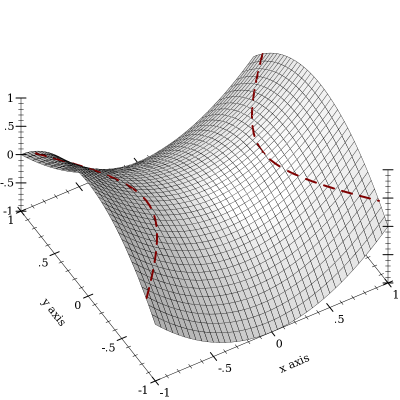(contours3d f [ x-min x-max y-min y-max #:z-min z-min #:z-max z-max #:samples samples #:levels levels #:colors colors #:widths widths #:styles styles #:alphas alphas #:label label]) → renderer3d?
f : (real? real? . -> . real?)
x-min : (or/c rational? #f) = #f
x-max : (or/c rational? #f) = #f
y-min : (or/c rational? #f) = #f
y-max : (or/c rational? #f) = #f
z-min : (or/c rational? #f) = #f
z-max : (or/c rational? #f) = #f
samples : (and/c exact-integer? (>=/c 2)) = (plot3d-samples)
 levels : (or/c 'auto exact-positive-integer? (listof real?)) = (contour-levels)
colors : (plot-colors/c (listof real?)) = (contour-colors)
widths : (pen-widths/c (listof real?)) = (contour-widths)
styles : (plot-pen-styles/c (listof real?)) = (contour-styles)
alphas : (alphas/c (listof real?)) = (contour-alphas)
label : (or/c string? #f) = #f
Returns a renderer that plots contour lines on the surface of a function.

The appearance keyword arguments are interpreted identically to the appearance keyword arguments to contours. In particular, when levels is 'auto, contour values correspond precisely to z axis ticks.

For example,
 > (plot3d (contours3d (λ (x y) (+ (sqr x) (sqr y))) -1.1 1.1 -1.1 1.1 #:label "z = x^2 + y^2"))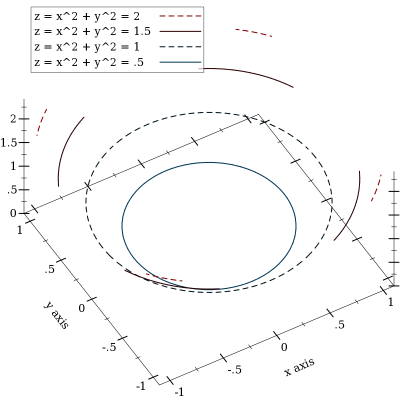(contour-intervals3d f [ x-min x-max y-min y-max #:z-min z-min #:z-max z-max #:samples samples #:levels levels #:colors colors #:styles styles #:line-colors line-colors #:line-widths line-widths #:line-styles line-styles #:contour-colors contour-colors #:contour-widths contour-widths #:contour-styles contour-styles #:alphas alphas #:label label])
renderer3d?
f : (real? real? . -> . real?)
x-min : (or/c rational? #f) = #f
x-max : (or/c rational? #f) = #f
y-min : (or/c rational? #f) = #f
y-max : (or/c rational? #f) = #f
z-min : (or/c rational? #f) = #f
z-max : (or/c rational? #f) = #f
samples : (and/c exact-integer? (>=/c 2)) = (plot3d-samples)
 levels : (or/c 'auto exact-positive-integer? (listof real?)) = (contour-levels)
 colors : (plot-colors/c (listof ivl?)) = (contour-interval-colors)
 styles : (plot-brush-styles/c (listof ivl?)) = (contour-interval-styles)
 line-colors : (plot-colors/c (listof ivl?)) = (contour-interval-line-colors)
 line-widths : (pen-widths/c (listof ivl?)) = (contour-interval-line-widths)
 line-styles : (plot-pen-styles/c (listof ivl?)) = (contour-interval-line-styles)
 contour-colors : (plot-colors/c (listof real?)) = (contour-colors)
 contour-widths : (pen-widths/c (listof real?)) = (contour-widths)
 contour-styles : (plot-pen-styles/c (listof real?)) = (contour-styles)
alphas : (alphas/c (listof ivl?)) = (contour-interval-alphas)
label : (or/c string? #f) = #f
Returns a renderer that plots contour intervals and contour lines on the surface of a function. The appearance keyword arguments are interpreted identically to the appearance keyword arguments to contour-intervals.

For example,
 > (plot3d (contour-intervals3d (λ (x y) (+ (sqr x) (sqr y))) -1.1 1.1 -1.1 1.1 #:label "z = x^2 + y^2"))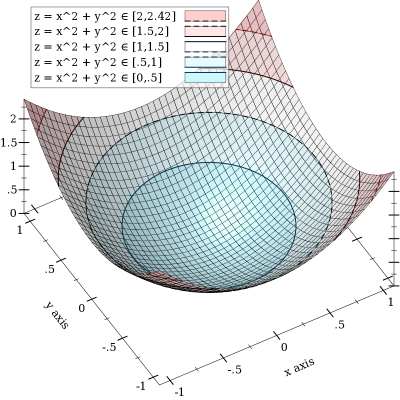#### 5.63D Isosurface Renderers

 (isosurface3d f d [ x-min x-max y-min y-max z-min z-max #:samples samples #:color color #:style style #:line-color line-color #:line-width line-width #:line-style line-style #:alpha alpha #:label label]) → renderer3d?
f : (real? real? real? . -> . real?)
d : rational?
x-min : (or/c rational? #f) = #f
x-max : (or/c rational? #f) = #f
y-min : (or/c rational? #f) = #f
y-max : (or/c rational? #f) = #f
z-min : (or/c rational? #f) = #f
z-max : (or/c rational? #f) = #f
samples : (and/c exact-integer? (>=/c 2)) = (plot3d-samples)
color : plot-color/c = (surface-color)
style : plot-brush-style/c = (surface-style)
line-color : plot-color/c = (surface-line-color)
line-width : (>=/c 0) = (surface-line-width)
line-style : plot-pen-style/c = (surface-line-style)
alpha : (real-in 0 1) = (surface-alpha)
label : (or/c string? #f) = #f
Returns a renderer that plots the surface of constant output value of the function f. The argument d is the constant value.

For example, a sphere is all the points in which the Euclidean distance function returns the sphere’s radius:
 > (plot3d (isosurface3d (λ (x y z) (sqrt (+ (sqr x) (sqr y) (sqr z)))) 1 -1 1 -1 1 -1 1) #:altitude 25)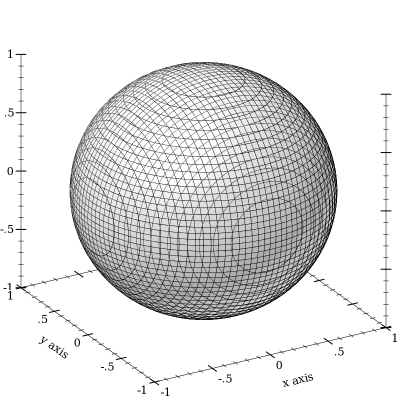(isosurfaces3d f [ x-min x-max y-min y-max z-min z-max #:d-min d-min #:d-max d-max #:samples samples #:levels levels #:colors colors #:styles styles #:line-colors line-colors #:line-widths line-widths #:line-styles line-styles #:alphas alphas #:label label]) → renderer3d?
f : (real? real? real? . -> . real?)
x-min : (or/c rational? #f) = #f
x-max : (or/c rational? #f) = #f
y-min : (or/c rational? #f) = #f
y-max : (or/c rational? #f) = #f
z-min : (or/c rational? #f) = #f
z-max : (or/c rational? #f) = #f
d-min : (or/c rational? #f) = #f
d-max : (or/c rational? #f) = #f
samples : (and/c exact-integer? (>=/c 2)) = (plot3d-samples)
 levels : (or/c 'auto exact-positive-integer? (listof real?)) = (isosurface-levels)
colors : (plot-colors/c (listof real?)) = (isosurface-colors)
 styles : (plot-brush-styles/c (listof real?)) = (isosurface-styles)
 line-colors : (plot-colors/c (listof real?)) = (isosurface-line-colors)
 line-widths : (pen-widths/c (listof real?)) = (isosurface-line-widths)
 line-styles : (plot-pen-styles/c (listof real?)) = (isosurface-line-styles)
alphas : (alphas/c (listof real?)) = (isosurface-alphas)
label : (or/c string? #f) = #f
Returns a renderer that plots multiple isosurfaces. The appearance keyword arguments are interpreted similarly to those of contours.

Use this to visualize functions from three inputs to one output; for example:
> (define (saddle x y z) (- (sqr x) (* 1/2 (+ (sqr y) (sqr z)))))
 > (plot3d (isosurfaces3d saddle #:d-min -1 #:d-max 1 #:label "") #:x-min -2 #:x-max 2 #:y-min -2 #:y-max 2 #:z-min -2 #:z-max 2)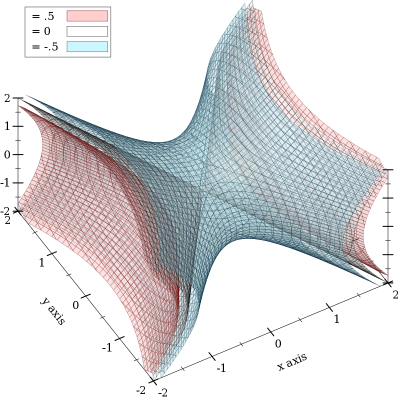If it helps, think of the output of f as a density or charge.

#### 5.73D Rectangle Renderers

 (rectangles3d rects [ #:x-min x-min #:x-max x-max #:y-min y-min #:y-max y-max #:z-min z-min #:z-max z-max #:color color #:style style #:line-color line-color #:line-width line-width #:line-style line-style #:alpha alpha #:label label]) → renderer3d?
rects : (listof (vector/c ivl? ivl? ivl?))
x-min : (or/c rational? #f) = #f
x-max : (or/c rational? #f) = #f
y-min : (or/c rational? #f) = #f
y-max : (or/c rational? #f) = #f
z-min : (or/c rational? #f) = #f
z-max : (or/c rational? #f) = #f
color : plot-color/c = (rectangle-color)
style : plot-brush-style/c = (rectangle-style)
line-color : plot-color/c = (rectangle-line-color)
line-width : (>=/c 0) = (rectangle3d-line-width)
line-style : plot-pen-style/c = (rectangle-line-style)
alpha : (real-in 0 1) = (rectangle-alpha)
label : (or/c string? #f) = #f
Returns a renderer that draws rectangles.

This can be used to draw histograms; for example,
> (require (only-in plot/utils bounds->intervals linear-seq))
> (define (norm2 x y) (exp (* -1/2 (+ (sqr (- x 5)) (sqr y)))))
> (define x-ivls (bounds->intervals (linear-seq 2 8 16)))
> (define y-ivls (bounds->intervals (linear-seq -5 5 16)))
> (define x-mids (linear-seq 2 8 15 #:start? #f #:end? #f))
> (define y-mids (linear-seq -5 5 15 #:start? #f #:end? #f))
 > (plot3d (rectangles3d (append* (for/list ([y-ivl  (in-list y-ivls)] [y  (in-list y-mids)]) (for/list ([x-ivl  (in-list x-ivls)] [x  (in-list x-mids)]) (define z (norm2 x y)) (vector x-ivl y-ivl (ivl 0 z))))) #:alpha 3/4 #:label "Appx. 2D Normal"))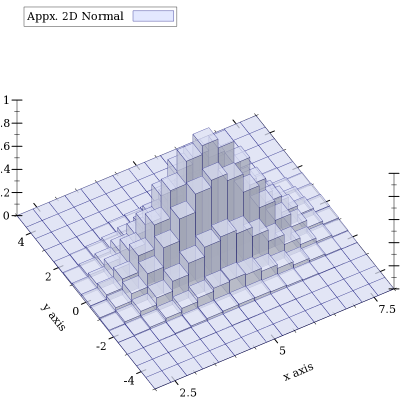(discrete-histogram3d cat-vals [ #:x-min x-min #:x-max x-max #:y-min y-min #:y-max y-max #:z-min z-min #:z-max z-max #:gap gap #:color color #:style style #:line-color line-color #:line-width line-width #:line-style line-style #:alpha alpha #:label label #:x-far-ticks? x-far-ticks? #:y-far-ticks? y-far-ticks?])
renderer3d?
cat-vals : (listof (vector/c any/c any/c (or/c real? ivl? #f)))
x-min : (or/c rational? #f) = 0
x-max : (or/c rational? #f) = #f
y-min : (or/c rational? #f) = 0
y-max : (or/c rational? #f) = #f
z-min : (or/c rational? #f) = 0
z-max : (or/c rational? #f) = #f
gap : (real-in 0 1) = (discrete-histogram-gap)
color : plot-color/c = (rectangle-color)
style : plot-brush-style/c = (rectangle-style)
line-color : plot-color/c = (rectangle-line-color)
line-width : (>=/c 0) = (rectangle3d-line-width)
line-style : plot-pen-style/c = (rectangle-line-style)
alpha : (real-in 0 1) = (rectangle-alpha)
label : (or/c string? #f) = #f
x-far-ticks? : boolean? = #f
y-far-ticks? : boolean? = #f
Returns a renderer that draws discrete histograms on a two-valued domain.

Missing pairs are not drawn; for example,
 > (plot3d (discrete-histogram3d '(#(a a 1) #(a b 2) #(b b 3)) #:label "Missing (b,a)" #:color 4 #:line-color 4))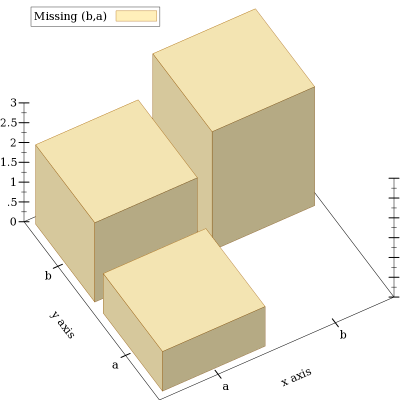(stacked-histogram3d cat-vals [ #:x-min x-min #:x-max x-max #:y-min y-min #:y-max y-max #:z-min z-min #:z-max z-max #:gap gap #:colors colors #:styles styles #:line-colors line-colors #:line-widths line-widths #:line-styles line-styles #:alphas alphas #:labels labels #:x-far-ticks? x-far-ticks? #:y-far-ticks? y-far-ticks?])
(listof renderer3d?)
cat-vals : (listof (vector/c any/c any/c (listof real?)))
x-min : (or/c rational? #f) = 0
x-max : (or/c rational? #f) = #f
y-min : (or/c rational? #f) = 0
y-max : (or/c rational? #f) = #f
z-min : (or/c rational? #f) = 0
z-max : (or/c rational? #f) = #f
gap : (real-in 0 1) = (discrete-histogram-gap)
colors : (plot-colors/c nat/c) = (stacked-histogram-colors)
 styles : (plot-brush-styles/c nat/c) = (stacked-histogram-styles)
 line-colors : (plot-colors/c nat/c) = (stacked-histogram-line-colors)
 line-widths : (pen-widths/c nat/c) = (stacked-histogram-line-widths)
 line-styles : (plot-pen-styles/c nat/c) = (stacked-histogram-line-styles)
alphas : (alphas/c nat/c) = (stacked-histogram-alphas)
labels : (labels/c nat/c) = '(#f)
x-far-ticks? : boolean? = #f
y-far-ticks? : boolean? = #f
Returns a renderer that draws a stacked histogram. Think of it as a version of discrete-histogram that allows multiple values to be specified for each pair of categories.

Examples:

> (define data '(#(a a (1 1 1)) #(a b (1.5 3)) #(b b ()) #(b a (1/2))))
 > (plot3d (stacked-histogram3d data #:labels '("Red" #f "Blue") #:alphas '(2/3 1 2/3)))